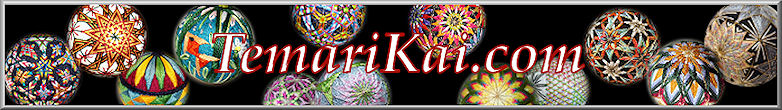#Geometry Basics for Temari

Let's be clear: no one needs to be a math major, or even conjure up that stuff from the required school math classes, in order to thoroughly enjoy making temari. While you certainly do not need to know, understand or use any of this geometry review, somewhere along the line when you progress and get into it, you may find some of these basic definitions, formulas and conversions come in handy.

Basic and Circular geometry:

Angle: the distance measured in degrees between two lines intersecting at a point.

Circumference: distance around the fullest part of the ball (abbreviated C)

Degrees in a circle: 360. If you move in a full circle around the center you travel 360 degrees.
Degrees in a line: 180. If you move from one end of a straight line to the other you travel 180 degrees.

Diameter: distance through the fullest part of a circle/ball (abbreviated D)
Radius: one half of the diameter (abbreviated r)

Pi = 3.14 (there is a value in geometry call Pi. It is a constant that makes all this work. Don't try to understand it; just use it.)

Formulas:

Diameter = r x 2 (radius times 2)
Circumference of a circle = D(Pi), or, D times Pi;  or (r x 2)D  which is radius times two, then multiply by Pi.

Conversions:

In general you will find that even if you use English/Impirical (inches, feet and yards) as your measuring values in life, using metric measurements of millimeters and centimeters is much easier, and really a must, for temari. Metric measurements are on a base of ten - you can measure, divide that measurement by any number and use the result directly. For example - if you want to divide a ball into fifths, and you are using inches with a circumference of say, 4.75 inches, you must divide 4.75 by 5, with the result being 0.95 inch. First, there is no easy way to measure 0.95 inches; inches are usually talked about in quarters, eighths, sixteenths; not tenths and hundredths. So, the first task is to figure out what 0.95 inch is on the ruler. Then, you will add 0.95 repeatedly to itself four times to get the segment distances around the ball: 0.95, 1.9, 2.85, 3.8, 4.75; this means in addition to figuring out what 0.95 inch is, you also need to figure out what 1.9, 2.85, and 3.8 inches are on the ruler, that is usually marked off in 1/8's or 1/16's. Ready to give up? That's why metric measuring is used in temari marking.

Metric measurements are based on 10, so it makes life easy (so is a calculator, which is why this all fits together so nicely). Metric rulers and tape measures are divided in tenths; that is how the metric system is based. All you need to remember is that there are 10 mm in 1 cm. Lets say the mari is 23.5cm in circumference. Divide that by 5, get 4.7. Now all you need to do is measure off segments of 4.7 cm around the strip to have five equal sections.

In another example, let's say that the distance you need to repeat is 0.8cm; remembering that the conversion between cm and mm is 10, this 0.8cm is also equal to 8mm. Cool, huh? Try doing something like that with inches and feet. Virtually all basic rulers and tape measures are English on one side and Metric on the other. Just start using the metric side.

1 Centimeter  (abbreviated cm) = 10 millimeters (abbreviated mm).

To convert from mm to cm, divide by 10.
To convert from cm to mm, multiply by 10.

For the diehards,

One cm = 0.3937 inches;
One mm= 0.03937 inches.
One inch = 2.54cm or 25.4mm

Remember that the standard for temari making is to refer to mari size by using circumference measured in cm. There is a conversion table available if needed, but the best thing is to get used to the standard and use it, and use metric measurements when discussing temari designs and techniques.